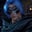Related Tags

communitycreator
python

# How to find the average of a list in PythonGutha Vamsi Krishna

### Problem statement

Given a list of numbers, find the average.

The average is the sum of elements divided by the number of elements.

### Example

• Input: [1, 2, 3, 4, 5, 6, 7, 8,9]

• Output: 5.0

#### Explanation

• Sum of elements = 1+2+3+4+5+6+7+8+9 = 45

• Number of elements = 9

• Average = $\frac{45}{9}$ = 5.0

### Solution

We can solve this problem with the following methods:

• The sum() method
• The reduce() and lambda methods
• The mean() method

### The sum() method

We will use the sum() and len() methods to find the average.

• sum() is used to find the sum of elements in the list.

• len() is used to find the number of elements in the list.

In the code snippet below:

• In line 1, we initialize the list list_of_numbers with [1,2,3,4,5,6,7,8,9].

• In line 5, we divide the sum of the elements by the number of elements to find the average.

• In line 8, we print the average to the console.

#initializing the list with numbers
list_of_numbers = [1,2,3,4,5,6,7,8,9]

#find average
avg = sum(list_of_numbers) / len(list_of_numbers)

#print average
print(avg)


### The reduce() and lambda methods

We use reduce() and lambda to calculate the sum of the elements in the list.

The following code snippet is the same as above, except for line 7, where we calculate the sum with reduce() and lambda.

from functools import reduce

#initializing the list with numbers
list_of_numbers = [1,2,3,4,5,6,7,8,9]

#find average
avg = reduce(lambda a, b: a + b, list_of_numbers) / len(list_of_numbers)

#print average
print(avg)

### The mean() method

We can use Python’s built-in mean() function to calculate the average of a list.

The following code snippet is the same as above, except for line 7, where we can find the average directly with the mean() method.

from statistics import mean

#initializing the list with numbers
list_of_numbers = [1,2,3,4,5,6,7,8,9]

#find average
avg = mean(list_of_numbers)

#print average
print(avg)

RELATED TAGS

communitycreator
python

CONTRIBUTORGutha Vamsi Krishna
RELATED COURSES

View all Courses

Keep Exploring

Learn in-demand tech skills in half the time